# Adversarial Inputs Against Deep Learning

Source: Deep Learning on Medium

In recent years, deep learning models have achieved state-of-the-art performance in a variety of areas, notably in the areas of computer vision and natural language processing. However, it has been discovered that these models are easily fooled by adversarial inputs — that is, attackers can make small but specific changes to typical inputs that cause incorrect model predictions. In this blog post, we attempt to explain what adversarial inputs are, why they exist, and how we can defend against them. We also take a look at some real-world applications of deep learning that are affected by adversarial inputs and discuss their practical implications. This blog post is written for the layman and does not require background knowledge of deep learning or adversarial machine learning.

### The problem of adversarial inputs

Consider the image below: is it a stop sign? To the human eye, even with a few added markings, it is indeed a stop sign. Now what if a deep neural network was responsible for determining what the sign is? Well, deep neural networks are already a crucial part of autonomous vehicle technology, and in many cases are responsible for deciphering the images taken by the powerful cameras of an autonomous vehicle. And it turns out that researchers have discovered that the minor change to the stop sign pictured below leads a deep neural network to identify it as a “Speed Limit 45” sign!?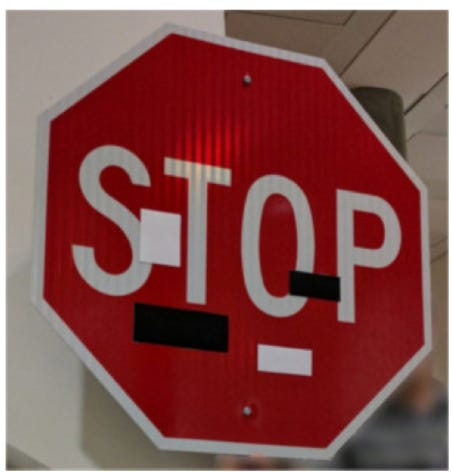Adversarial Stop Sign. Image Source: https://arxiv.org/pdf/1707.08945.pdf

Let’s consider another image, this time of a number. It is easy for the human eye to see that the number in the image is 7. Once again, as we show in the next section, we are able to fool a deep neural network into predicting the number in the image as a 2.Image of the digit 7.

The two examples above illustrate the problem of adversarial inputs — inputs that look typical to the human eye, but which are perturbed in a small fashion, can easily fool deep neural networks into making incorrect predictions.

At this time, one may wonder what causes neural networks to be fooled by the above inputs. The short answer is that due to the design of neural networks, an adversary that has access to the neural network can figure out what changes to a typical input will lead to an incorrect prediction. We try to provide an intuitive explanation of how this fooling is carried out by an adversary in the section titled “Why do adversarial inputs exist?”. In fact, it has also been shown that an adversary can create adversarial inputs for neural networks without having complete access to the network. As we will discuss later, this weakness can have damaging consequences when neural networks are deployed in real world applications such as autonomous driving, speech recognition, and malware detection. Readers who are more interested in the practical implications of these issues can jump to the section titled “Practical threats due to adversarial inputs”.

Given the above, it is only natural to ask whether such adversarial inputs exist naturally, without the intervention of an adversary. For instance, is it possible for a autonomous vehicle to see a stop sign in a thunderstorm and classify it as a “Speed Limit 65” sign? What are the implications of such mistakes? In order to tackle the above questions, we must first understand what adversarial inputs are and how they fool neural networks.

### What are adversarial inputs?

Adversarial inputs lack a precise definition as their defining properties vary from one problem domain to another. For example, in the problem of image classification, given an image, one would consider an adversarial input to be an image that looks very similar to the given image but is classified incorrectly. Here, similarity may be measured in terms of how close the two images appear to a human observer or by some other mathematical similarity measure. In a malware classification problem, an adversarial binary may be a binary that preserves the semantic functionality of the original (malicious) binary but is misclassified as a clean binary by adding a few carefully chosen bytes the original binary. In this case, the similarity between the two binaries can be measured by their functionality, which is identical. In this blog, we choose to illustrate the notion of adversarial inputs using the computer vision problem domain as it is the simplest to grasp.

Consider the MNIST handwritten digit classification problem, where the dataset consists of grayscale images of handwritten digits between 0–9 and the machine learning classification task is to correctly label the digit on a given input image. We provide a few examples of such images below.Original MNIST Images

We built and trained a neural network to classify the above images correctly (i.e. it assigned class labels: 2, 7, 9, 0, and 6 to the above images). Now examine the images below — they seem to be very similar to the images above — however each of these images was incorrectly classified by the same neural network (i.e. it assigned class labels: 4, 2, 3, 9, and 1 to the images below) . That is, we were able to construct adversarial images from the original images that look almost identical to the human eye but fooled the neural network into making incorrect predictions.Adversarial Images

In order to understand how these adversarial inputs are created and why it is possible to create them, it is important to understand how neural networks make predictions. Before we are able to explain the prediction mechanism of neural networks, we must provide a brief introduction to them.

### What are neural networks?

A neural network is a machine learning algorithm that is modeled loosely on the human brain. As the name suggests, a neural network consists of a network of nodes (neurons) organized in a layer-wise manner, where a layer is simply a collection of neurons. For instance, a 5 layer neural network with 100 neurons in each layer means that the neural network consists of 5 different layers (explained further below) where each layer consists of 100 nodes (neurons). As an aside on terminology: the more layers a neural network consists of, the “deeper” it is, which is why the term “neural network” and “deep neural network” are used interchangeably. The sub-field of machine learning that uses neural networks (or deep neural networks) is referred to as deep learning. For a more complete introduction to neural networks we refer the reader to the easily accessible materials on deep learning and convolutional neural networks.

Below is an image taken from these materials depicting the layer-wise structure of neural networks. The image depicts a 3 layer neural network with 4 nodes (neurons) in the first layer, 4 nodes (neurons) in the second layer and 1 node(neuron) in the last layer (the reader should ignore the use of “hidden” layer and “output” layer for now). The input layer is not considered to be a part of the neural network, it is simply a node based depiction of the input (in the image below, it consists of 3 nodes). Each node in the network holds a number. For instance, if the input is a 28 x 28 gray scale image, then the value of each pixel of the image will be held in exactly one node of the 784 nodes in the input layer. The arrows connecting a node to every node in the layer to the right means that the value from a given node will flow forward to all the nodes in the layer to the right of it. Hence, a neural network makes predictions by pushing the values from the input layer through the network and finally through the output layer which is the answer to a given problem. For instance, a network might take the image of a cat in the input layer and output 0.99 in the output layer, which could be the probability the network assigns to the input image being a cat.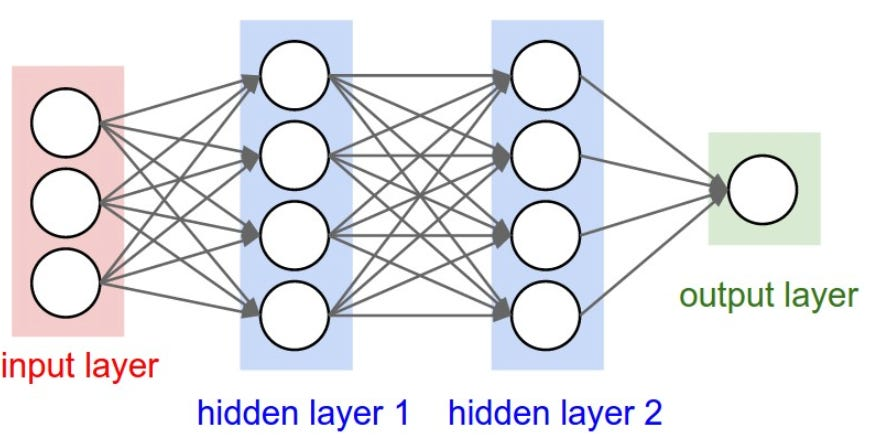Structure of a 3 layer neural network. Image source: http://cs231n.github.io/neural-networks-1/

One of the primary reasons behind the success of neural networks [AG1] is that they only need labeled data in order to learn to solve a particular task. This means that if we provide a neural network with enough images of cats and dogs where each cat image is labeled as a cat and each dog image is labeled as a dog, the network will be able to itself learn the complex relationships required to tell if a new image is a cat or a dog. With this brief introduction to neural networks, we can now proceed to understand how they make predictions.

### How do deep neural networks make predictions?

CClassifiction is the problem of assigning a class label to a given input, i.e. given an input x, assign it to a class k. For example, in the MNIST handwritten digit classification problem, given an image of a handwritten digit, the classification task is to assign it to a class between 0–9. For most problem domains, the input x belongs to some subset of a finite dimensional Euclidean space, which is commonly referred to as the input space. Any input is a point in this input space, and a deep neural network then divides this space into a small enclosed regions and assigns a class label to each region. One can think of each layer of the neural network as learning successively more and more about the input space, and then successively dividing the input space up into more and more regions. At the output layer, each enclosed region is assigned a particular class label.

This means that every input that lies in a particular enclosed region will be assigned the same class label by the neural network. The lines or curves that divide this input space form what is called the decision boundary. The number of lines or curves used by a neural network to divide the input space is arbitrary as it depends on the architecture of the neural network and is not important for this discussion (more specifically, it depends on the number of layers, and the number of neurons per layer). We illustrate this gradual process with a 3 layer neural network as it divides a 2 dimensional input space and forms its decision boundary.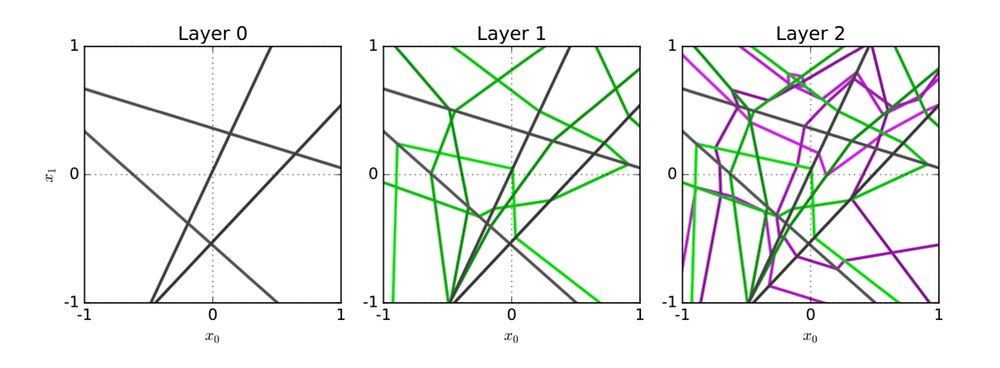2D Input space being divided gradually by each layer. Image source: https://arxiv.org/pdf/1606.05336.pdf

The first layer (Layer 0) of this neural network uses 4 lines to divide the input space, resulting in 9 enclosed regions, the second layer (Layer 1) adds more lines per enclosed region to further divide each enclosed region into more enclosed regions, and the third layer (Layer 2) further divides each enclosed region in a similar manner and also assigns a class label to each enclosed region in the input space. The final classification of the neural network is then based on the class labels that the final layer (third layer in the image above) assigns to each enclosed region. That is, after the action of the final layer, every enclosed region is assigned a class label and any input belonging to the same enclosed region will be assigned the same class label by the neural network. Since the neural network uses this process to divide the entire input space, it is able to assign a class label to any input. We now explain how this leads to the creation of adversarial inputs.

### Why do adversarial inputs exist?

Albeit very powerful, the above process of dividing the input space also makes the neural network vulnerable to adversarial inputs. This is because in many cases neighboring regions have different class labels (in fact this would be true in more cases than not — the reasons behind why neighboring regions may have the same class label are more technical and not crucial for this discussion). Therefore, an adversary can take an input belonging to an enclosed region with class label k (we assume k is the correct label), and change it by a very small amount such that the input is now shifted to a neighboring enclosed region with a different class label j. When this modified input is presented to the neural network, the network will classify it as belonging to class j. Therefore, the adversary has thereby changed the class label that will be predicted by the neural network from a correct one to an incorrect one. However, since the input was only changed by a small amount, it is still very similar to the original input causing the phenomenon of being adversarial. As a techinical aside, it is important to note here that this phenomenon arguably worsens in higher dimensional spaces as most of the points are concentrated on the decision boundary.

One may ask how an adversary knows what changes will cause the input to shift into the desired neighboring region? The short answer is that the adversary utilizes the knowledge of the neural network to craft the required adversarial changes. The long answer requires a deeper understanding neural networks, how they are trained, basic calculus, and optimization. Therefore, we leave it out as an exposition on that topic is not our goal. However, it has been shown that adversaries can also craft adversarial inputs without detailed knowledge of the targeted neural network as well. It is crucial to understand just how many of these regions can be created by neural networks in order to understand just how easy it is to craft adversarial inputs. To illustrate this process, we use the image below which shows how a 1 layer neural network with 1024 neurons divides 2 dimensional input space (we ignore projection details used by the authors for simplicity).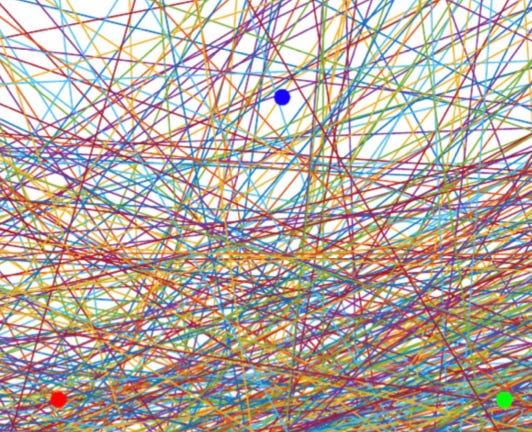2D Input Space divided by 1024 neurons in 1 Layer. Image Source: https://arxiv.org/pdf/1810.07481.pdf

One can see that for any enclosed regions, just how many other enclosed regions are neighbors. The authors also show three inputs belonging to three different classes (i.e. residing in three different regions with different labels). As can be seen, in order to change the label for any of the three inputs, only a small change is required as they are surrounded by numerous regions belonging to different classes.

This raises the question: just how susceptible neural networks are to such adversarial inputs? The answer varies and depends largely on this arrangement of lines or curves in the input space. Consider a neural network that is able to assign the correct class label to any input from the problem domain, can that neural network still be susceptible to adversarial inputs? Yes. Depending on the manner in which the network has divided up the input space, there may still exist regions with a class label k (which is the correct label), that are neighbors of regions with a different class label. Hence, an adversary simply has to perturb the original input in a manner that shifts it into one of the neighboring regions with a different class label in order to fool the network.

The above phenomenon has far reaching security implications in tasks such as facial recognition, malware classification, autonomous driving, financial predictions, and many more. A malicious actor could use this knowledge to masquerade as someone else, modify predictions on business-critical systems, etc. Given the critical impact of this problem, it is natural to ask how we can defend against it.

### How can we defend against adversarial inputs?

As explained above, adversarial inputs are crafted by making small changes to the original input. However, these small changes in the input lead to large changes in the neural network output (i.e. network predictions change). Therefore, one natural approach to defend against adversarial inputs would be to ensure that network outputs do not change by a large amount if an attacker makes small changes to the input. This seemingly simple goal however is difficult to accomplish in practice. Most methods attempt to achieve this goal by penalizing the neural network during its training phase to control the amount of change in network output with respect to small changes in the input (we do not explain the details of how this penalization occurs as it requires a deeper understanding of neural networks and the details may take away from the primary point). Although this solution hardens the neural network against adversarial inputs (requires a larger change in the original input in order to fool the network), it does not solve the problem completely. Due to the manner in which a neural network divides up the input space, it is still possible to create adversarial inputs.

Another provable defense against adversarial inputs is to manipulate the decision boundary around the training inputs of the neural network. That is, we move the decision boundary farther away from the training points, hence requiring a larger change to the original input to fool the network. This is illustrated in the image below. Once again, this is a limited defense: it is only applicable to the inputs that the network trained on, and it does not extend to every new test input.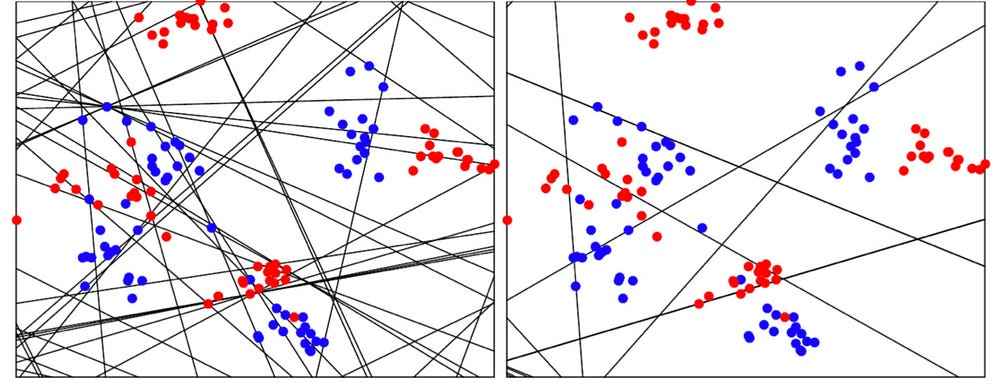Left: Original Decision Boundary. Right: Decision boundary after defense is applied. Image source: https://arxiv.org/pdf/1704.08847.pdf

Other defenses use variations of the above ideas in order to make it more difficult for the adversary to fool the network. However, there do not exist any defenses that guarantee protection against adversarial inputs for general deep neural networks. As discussed previously, this is largely due to the manner in which neural networks divide up the input space. Since neural networks have become widely adopted to solve practical problems such as facial recognition, autonomous driving, etc., we must examine the impact of adversarial inputs in such applications.

### Practical threats due to adversarial inputs

Due to their superior efficacy and data driven learning, neural networks are extremely popular in practical applications. We examine some real world applications of deep learning and how they are affected by adversarial inputs. We examine some real world applications of deep learning and how they are affected by adversarial inputs.

Autonomous driving technology utilizes deep neural networks for various tasks such as object detection and scene segmentation (determining which class each pixel of an image belongs to). It has been shown that neural networks responsible for segmenting a perceived image can be fooled into an incorrect segmentation of a scene, for example: seeing trucks or cars where there are none, or not seeing a pedestrian where there is one. Another line of work has shown that adversarial inputs also exist in the real world (i.e. it is possible to create an adversarial input, print it out and fool a neural network that looks at this picture). Combining these two properties, one can see that an autonomous driving system that only utilizes deep learning for low level perception may be easily fooled by an adversary. Even without the presence of an adversary, it is crucial to consider how autonomous vehicles perform in extreme conditions (or corner cases).

Another powerful application of deep learning is in speech recognition-based assistants such as Siri, Alexa, and Google Now. These are also vulnerable to adversarial inputs as it has been shown that one can construct inaudible commands that fool these voice assistants into completing simple tasks such as initiating a Face-time call. That is, an adversary can create voice signals which are inaudible to a human, but that fool a voice assistant into completing a particular task. Once again, given the ability of the voice assistant and the sensitivity of the task, this is a critical security issue for such voice recognition systems.

Finally, consider the case of malware classification where a malware detection system examines a file and decides whether it is malicious or not. It has been shown that one can modify a malicious file in a manner that maintains the malicious functionality of the file but fools a neural network based malware detection system into classifying the file as being non-malicious . One can see that the security implications of the vulnerability can be far reaching since a malicious file may be able evade detection and compromise a host.

### Conclusion

As shown in this article, adversarial inputs pose a serious threat to deep learning systems. This problem becomes especially important given the large scale adoption of deep neural networks across a variety of domains. Since one of the primary causes of the adversarial phenomenon is the fundamental design of deep neural networks, there do not currently exist any general defenses to this problem and the discovery of such a defense seems unlikely. Hence, it is crucial to examine this problem from a theoretical perspective to formally understand the limits of deep neural networks against adversarial inputs. Even though there are no perfect defenses against adversarial inputs, practitioners currently deploying deep learning models, can try to use existing defenses that reduce the damage for specific network architectures and problem domains.